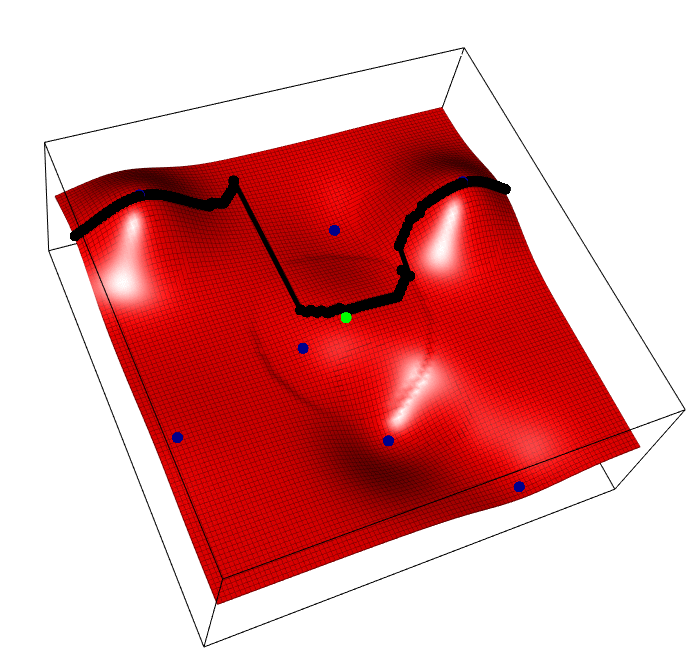# Continuous Multi-Task Bayesian Optimization with Correlation

2018, Oct 21
Standard Bayesian optimisation deals with finding the best input $$x \in X$$ to a stochastic noisy expensive black box function $$f(x)$$. If I had, say, 10 expensive black box functions all over the same input domain $$f_{1}(x),..., f_{10}(x)$$, surely I do not need to optimise all 10 independently?
We consider this problem and show that when given a range of objective functions (multiple tasks), optimizing one can speed up optimisation of the rest. Specifically collecting data (pick one $$f_{i}(x)$$, pick one $$x \in X$$, observe one $$y$$) accounting for how that new data point influences all functions can lead to significant performance improvement across all functions.The horizontal axis is the task parameter $$i$$, the depth/vertical axis the input for that task $$x$$, and we aim to find the best input for each task $$max_x f(i,x)$$, i.e. optimize each vertical slice. Blue points are observed points, the green point is one possible new point whose y value is random, and the black points show the predicted peak of each slice, that will change as a result of the new green addition.